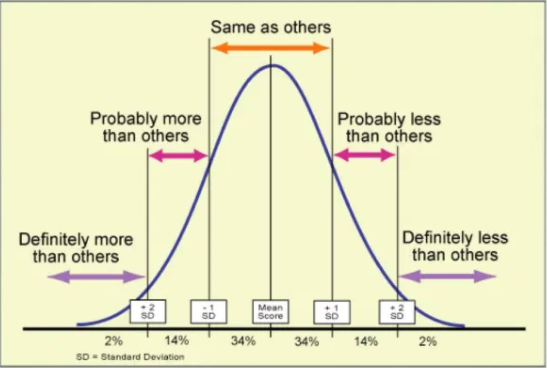# What is the difference between Average and Standard Deviation duration within Retreaver?

### Retreaver Standard Deviation Average Duration

What is the difference between Average and Standard Deviation duration within Retreaver?

###The Average Duration is the true average, but the Standard Deviation value looks at the duration by taking out the outliers that might skew the average (calls that are super short, or calls that are super long)

###The Standard Deviation value basically gives you an average, without the numbers being skewed by really short calls or really long calls. For example, if you have a ton of connection issues where the call lasts only 5 seconds long, those calls will bring down the average duration significantly, what the standard deviation does is exclude those values mathematically to give you a value without the outlier calls.

### How is Standard Deviation calculated?

Step 1:  Get the true average value.

Step 2:  Subtract the mean from actual duration, which will give you the value the call has to the mean. (if the call is 4mins long and the average is 3 the value will be -1).

Step 3: Square the distance from actual time to average ( the purpose for this is to remove the negative values and prevent negative values from cancelling out positive values).

Step 4: Add all those square values up and divide by the number of calls.

Step 5 : Take that ending value and square root it to remove the square we did in step 3 and that's how the outliers are not considered statistically.

0 out of 0 found this helpful